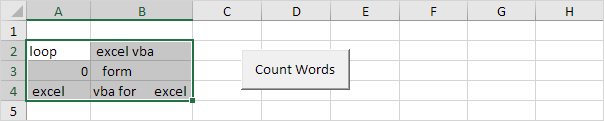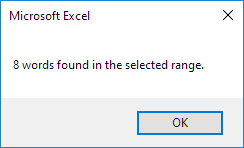# Count Words

Below we will look at a program in Excel VBA that counts the number of words in a selected range. One or more spaces are assumed to separate words.

Situation:1. First, we declare two Range objects and three variables. We call the Range objects rng and cell. One Integer variable we call cellWords, one Integer variable we call totalWords, and one String variable we call content.

Dim rng As Range, cell As Range
Dim cellWords As Integer, totalWords As Integer, content As String

2. We initialize the Range object rng with the selected range and the two variables of type Integer with value 0.

Set rng = Selection
cellWords = 0
totalWords = 0

3. We want to check each cell in a randomly selected range (this range can be of any size). In Excel VBA, you can use the For Each Next loop for this. Add the following code lines:

For Each cell In rng

Next cell

Note: rng and cell are randomly chosen here, you can use any names. Remember to refer to these names in the rest of your code.

4. Next, we determine for each cell in this range how many words it contains. To ignore a cell that contains a formula, add the following code line between For Each and Next (only if cell.HasFormula is false we continue).

If Not cell.HasFormula Then

End If

5. First, we write the content of the cell to the variable content. Next, we remove the spaces at the beginning and the end (if there are any). In Excel VBA, you can use the Trim function for this. For example, " excel vba" will be converted to "excel vba". Add the following code lines in your If statement.

content = cell.Value
content = Trim(content)

Note: the trim function in Excel VBA does not remove extra spaces between words, but that's OK in this example.

6. At this point, a cell can still be empty. If the cell is empty, we assign the value 0 to the variable cellWords. If not, it contains at least one word and we assign the value 1 to the variable cellWords. Add the following code lines in your If statement.

If content = "" Then
cellWords = 0
Else
cellWords = 1
End If

A cell can contain more than one word of course. That's exactly what we want to find out now. As an example we take: "excel vba". If a cell contains at least one space at this stage, it contains at least one more word. You can use the Instr function in Excel VBA to look for a space. Instr(content, " ") finds the position of the first space in content.

7. We will make use of the Do While Loop structure. Code placed between these words (at step 8, 9 and 10) will be repeated as long as the part after Do While is true. We want to repeat these steps as long as Instr(content, " ") > 0 is true (as long as content contains a space and thus more words). Add the Do While Loop in your If statement.

Do While InStr(content, " ") > 0

Loop

8. Next, we take the part of content starting at the position of the first space. We use the Mid function for this.

content = Mid(content, InStr(content, " "))

For example: Mid("excel vba", InStr("excel vba", " ")) will give " vba".

9. We trim the string again.

content = Trim(content)

Result: "vba"

10. We increment cellWords by 1.

cellWords = cellWords + 1

This Do While Loop will be repeated as long as content contains a space and thus more words. In our example, we exit the Do While Loop since "vba" does not contain a space anymore! Result: this cell contains 2 words.

11. After having checked one cell, we add cellWords to the variable totalWords. This code line should be placed outside the Do While Loop but in the If statement.

totalWords = totalWords + cellWords

The whole process starts again for the next cell until all cells have been checked.

12. Finally, we display the value of totalWords using a msgbox. This code line should be placed outside the For Each Next loop.

MsgBox totalWords & " words found in the selected range."

13. Test the program.

Result:Go to Next Chapter: Date and Time